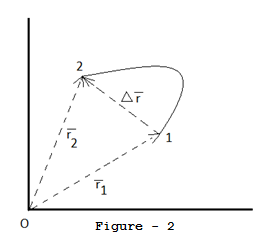### Distance and Displacement

From the name itself you will understand these two terms, “distance” and “displacement”. However, I will share with you some basic knowledge regarding those terms.
Imagine that you were at a place called “A” at time t1.If you have to go to the place “C” through the place “C” as shown in the figure 1.And if you reach at “C” at time t2. Ie, within the time interval t1 and t2 you covered the path “ABC” as in the figure 1 below.
Then, the total distance that you have covered in the interval t1 and t2 equals to the length of the path “ABC” is called distance. This can be expressed with a numerical value completely and so it is a scalar quantity.
When analyzing your path you have started from the place “A” and you reached at ”B”.ie,finally you have just moved from A to C. The straight distance between A and C is called displacement in the interval t1 and t2 (this has shown in the figure as dotted line).Without the initial and final positions, we cannot express this term completely.ie, Displacement is a vector quantity (means, needs the magnitude and direction).The direction of displacement is from initial position to final position.
Displacement can be zero, positive or negative.
In general, distance travelled between two points may not be equal to magnitude of the displacement between same points.If you know some basics of vector addition, you can solve the problems to find the displacement easily in a 3D space. Imagine a particle at the position “1” at time, t1. If the same particle is at position “2” at time,t2 as shown in the figure 2 below. Here, r1 is the position vector of “1” as in the figure. Similarly, r2 is the position vector of position “2”.Position vector is the vector with tail at origin and head directing to a position. r  is the displacement vector having direction from initial to final position(ie, from 1 to 2).
By vector addition we know that,  r1 + r = r2 .
So, r = r2 – r1.

ie, Displacement = final position vector – initial position vector.
Stay online for more from the team.simucentral